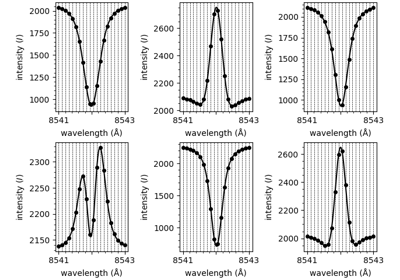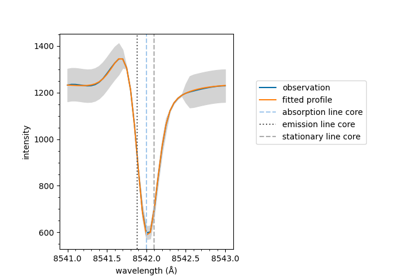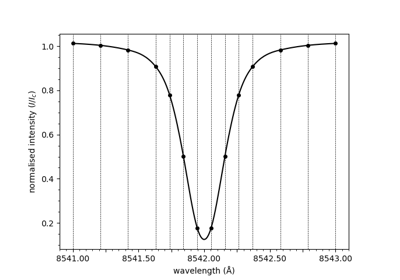# plot_spectrum

mcalf.visualisation.plot_spectrum(wavelengths, spectrum, normalised=True, smooth=True, ax=None)[source]

Plot a spectrum with the wavelength grid shown.

Intended for plotting the raw data.

Parameters:
• wavelengths (numpy.ndarray) – The x-axis values.

• spectrum (numpy.ndarray, length=n_wavelengths) – The y-axis values.

• normalised (bool, optional, default=True) – Whether to normalise the spectrum using the last three spectral points.

• smooth (bool, optional, default=True) – Whether to smooth the spectrum with a spline.

• ax (matplotlib.axes.Axes, optional, default=None) – Axes into which the fit will be plotted. Defaults to the current axis of the current figure.

Returns:

ax – Axes the lines are drawn on.

Return type:

matplotlib.axes.Axes

ExamplesUsing IBIS8542Model

Using IBIS8542ModelPlot a fitted spectrum

Plot a fitted spectrumPlot a spectrum

Plot a spectrum